Courses

Fill in the Blanks & True/False: Laws of Motion | JEE Advanced Notes | Study Physics 35 Years JEE Main & Advanced Past year Papers - JEE

JEE: Fill in the Blanks & True/False: Laws of Motion | JEE Advanced Notes | Study Physics 35 Years JEE Main & Advanced Past year Papers - JEE

The document Fill in the Blanks & True/False: Laws of Motion | JEE Advanced Notes | Study Physics 35 Years JEE Main & Advanced Past year Papers - JEE is a part of the JEE Course Physics 35 Years JEE Main & Advanced Past year Papers.
All you need of JEE at this link: JEE

Fill in the Blanks

Q.1. A block of mass 1 kg lies on a horizontal surface in a truck. The coefficient of static friction between the block and the surface is 0.6. If the acceleration of the truck is 5 m/s2, the frictional force acting on the block is ..... newtons.     (1984 - 2 Marks)

Ans. 5

Solution. As seen by the observer on the ground, the frictional force is responsible to move the mass with an acceleration of 5 m/s2.

Therefore, frictional force = m × a = 1 × 5 = 5 N.

Q.2. A uniform rod of length L and density ρ is being pulled along a smooth floor with a horizontal acceleration  α (see Fig.) The magnitude of the stress at the transverse crosssection through the mid- point of the rod is .........    (1993 - 1 Mark)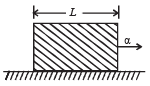Ans. ρLα/2

Solution. Let A be the area of cross-section of the rod.

Consider the back half portion of the rod.

Mass of half portion of the rod = ρAL/2

The force responsible for its acceleration is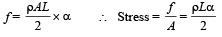True/False

Q.1. A rocket moves forward by pushing the surrounding air backwards.

Ans. F

Solution. KEY CONCEPT : The rocket moves forward when the exhaust gases are thrown backward.

Here exhaust gases thrown backwards is action and rocket moving forward is reaction.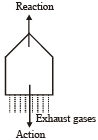Note : This phenomenon takes place in the absence of air as well.

Q.2. When a person walks on a rough surface, the frictional force exerted by the surface on the person is opposite to the direction of his motion.   (1981 - 2 Marks)

Ans. F

Solution. KEY CONCEPT : Friction force opposes the relative motion of the surface of contact.

When a person walks on a rough surface, the foot is the surface of contact. When he pushes the foot backward, the

motion of surface of contact tends to be backwards.

Therefore the frictional force will act forward (in the direction of motion of the person)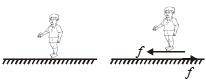Q.3. A simple pendulum with a bob of mass m swings with an angular amplitude of 40°. When its angular displacement is 20°, the tension in the string is greater than mg cos 20°. (1984 - 2 Marks)

Ans. T

Solution. As the angular amplitude of the pendulum is 40°, the bob will be in the mid of the equilibrium position and the extreme position as shown in the figure

Note : For equilibrium of the bob, T – mg cos 20° =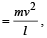where l is the length of the pendulum and is the velocity of the bob.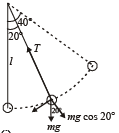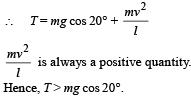Q.4. The pulley arrangements of Figs.  (a) and  (b) are identical. The mass of the rope is negligible. In (a) the mass m is lifted up by attaching a mass 2m to the other end of the rope. In (b) , m is lifted up by pulling the other end of the rope with a constant downward force F = 2 mg. The acceleration of m is the same in both cases                   (1984 - 2 Marks)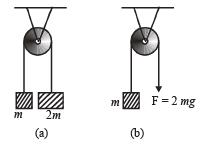Ans. F

Solution. Case (i) For mass m
T – mg = ma ... (i)
For mass 2m
2mg – T = 2ma ... (ii)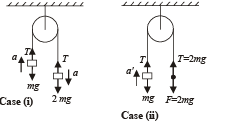From (i) and (ii) a = g/3
Case (ii) T – mg = ma'
2mg – mg = ma' [∴ T = 2mg]
∴ a' = g
Hence, a < a'

The document Fill in the Blanks & True/False: Laws of Motion | JEE Advanced Notes | Study Physics 35 Years JEE Main & Advanced Past year Papers - JEE is a part of the JEE Course Physics 35 Years JEE Main & Advanced Past year Papers.
All you need of JEE at this link: JEEUse Code STAYHOME200 and get INR 200 additional OFF Use Coupon Code

Top Courses for JEEPhysics 35 Years JEE Main & Advanced Past year Papers

88 docs|49 tests

Top Courses for JEETrack your progress, build streaks, highlight & save important lessons and more!

,

,

,

,

,

,

,

,

,

,

,

,

,

,

,

,

,

,

,

,

,

;